﻿﻿

# 利用pandas进行数据分组及可视化

kaggleTitanic数据集，给定了Titanic号邮轮的乘客的船舱等级（Pclass）、性别（Sex）、年龄（Age）、是否获救（Survived）等信息。希望能够用这些信息建立一个分类系统，来预测一个人是否会获救。

import numpy as np

import pandas as pd

import matplotlib.pyplot as plt

from pandas import DataFrame,Series

data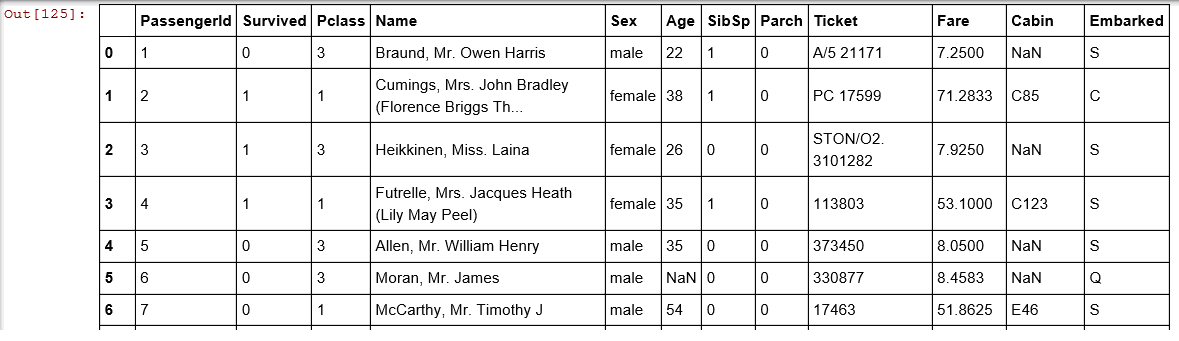data.info()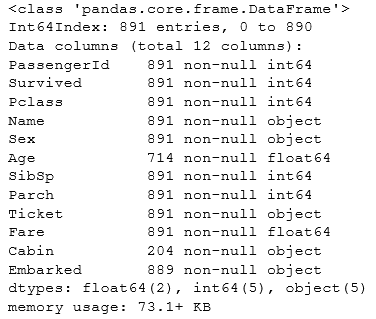teen1 = t[(t.Pclass == 1) &(t.Age<20)]['PassengerId'].count()

four1=t[(t.Pclass == 1) & (t.Age>20) & (t.Age < 40)]['PassengerId'].count()

six1=t[(t.Pclass == 1) & (t.Age >40)& (t.Age < 80)]['PassengerId'].count()

teen2 = t[(t.Pclass == 2) &(t.Age<20)]['PassengerId'].count()

four2=t[(t.Pclass == 2) & (t.Age>20) & (t.Age < 40)]['PassengerId'].count()

six2=t[(t.Pclass == 2) & (t.Age >40)& (t.Age < 80)]['PassengerId'].count()

teen3 = t[(t.Pclass == 3) & (t.Age<20)]['PassengerId'].count()

four3=t[(t.Pclass == 3) & (t.Age>20) & (t.Age < 40)]['PassengerId'].count()

six3=t[(t.Pclass == 3) & (t.Age >40)& (t.Age < 80)]['PassengerId'].count()

allteen = t[t.Age<20]['PassengerId'].count()

allfour=t[ (t.Age >20) & (t.Age < 40)]['PassengerId'].count()

allsix=t[ (t.Age >40 )& (t.Age <80)]['PassengerId'].count()

s=DataFrame({'all':[allteen,allfour,allsix],'P1':[teen1,four1,six1],'P2':[teen2,four2,six3],'P3':[teen3,four3,six3]},index=['0-20','20-40','40-'])

s.plot(kind='bar')

plt.show()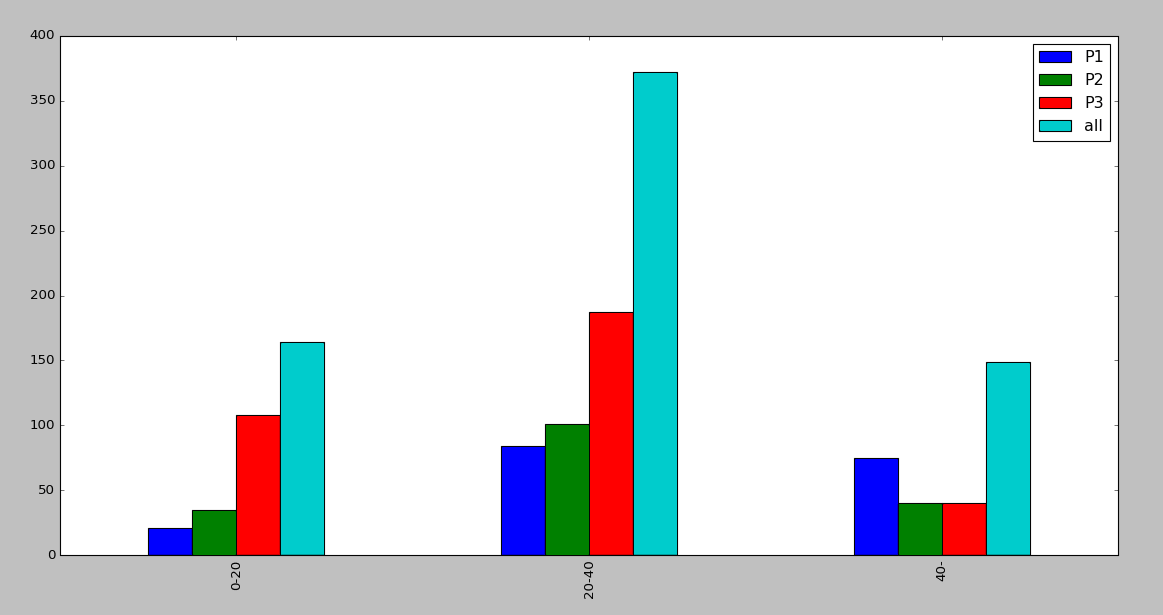def cla(n,lim):

return'[%.f,%.f)'%(lim*(n//lim),lim*(n//lim)+lim) # map function

addone = Series([cla(s,10) for s in t.Age])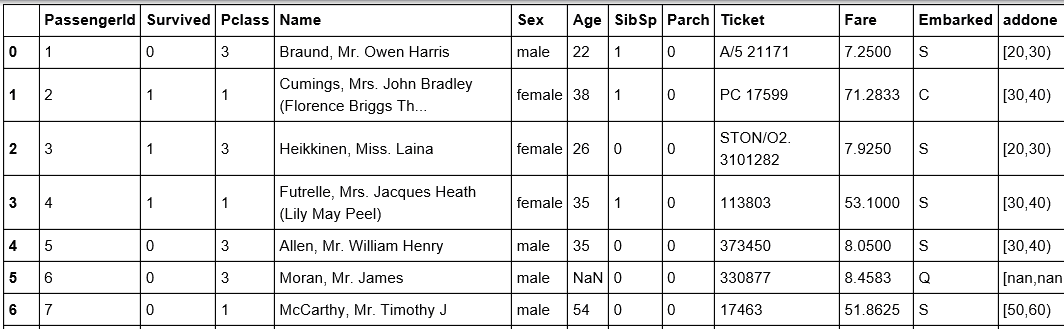groups = t.groupby(['Pclass','addone']).count()   # beautiful graph

groups是这样一个DataFrame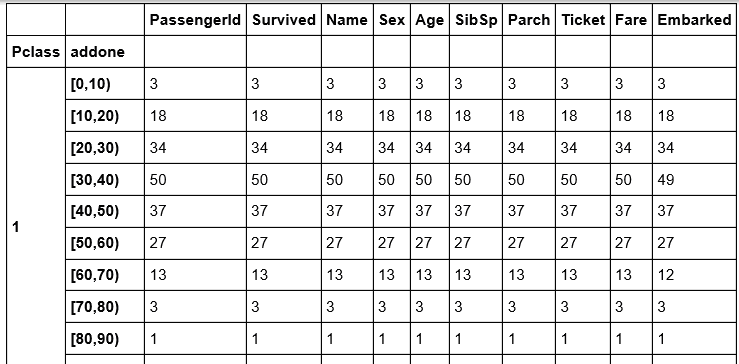groups['PassengerId'].plot('bar')

plt.show()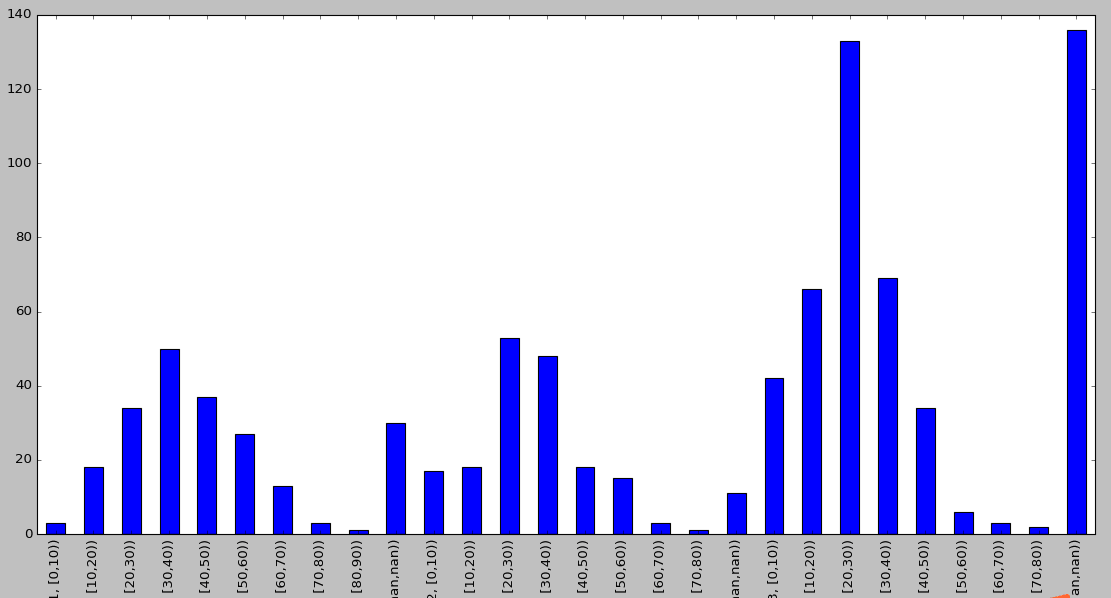tmp = groups['Name']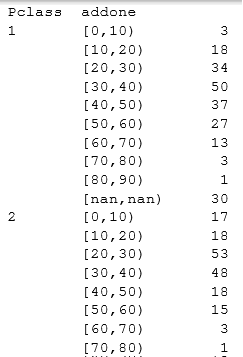tmp2 = tmp.unstack()

tmp2如下：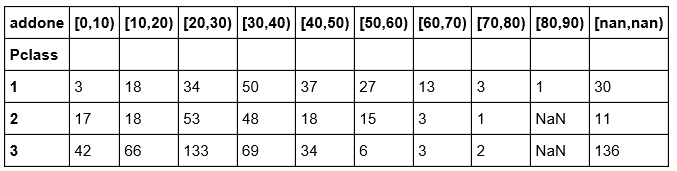tmp2.plot(kind='bar')

plt.show()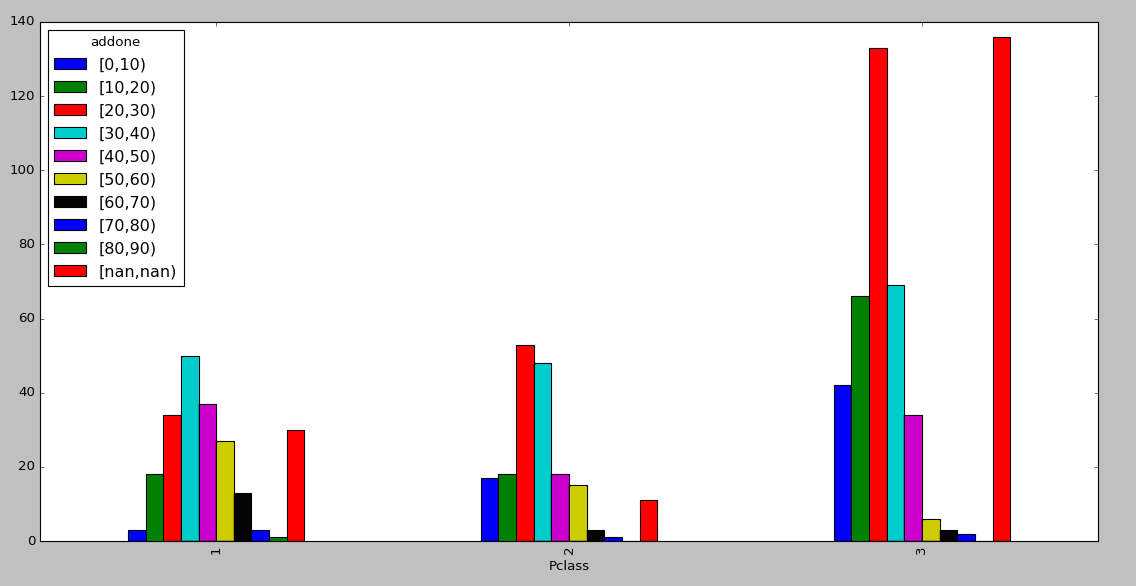(tmp2.T).plot(kind='bar')

plt.show()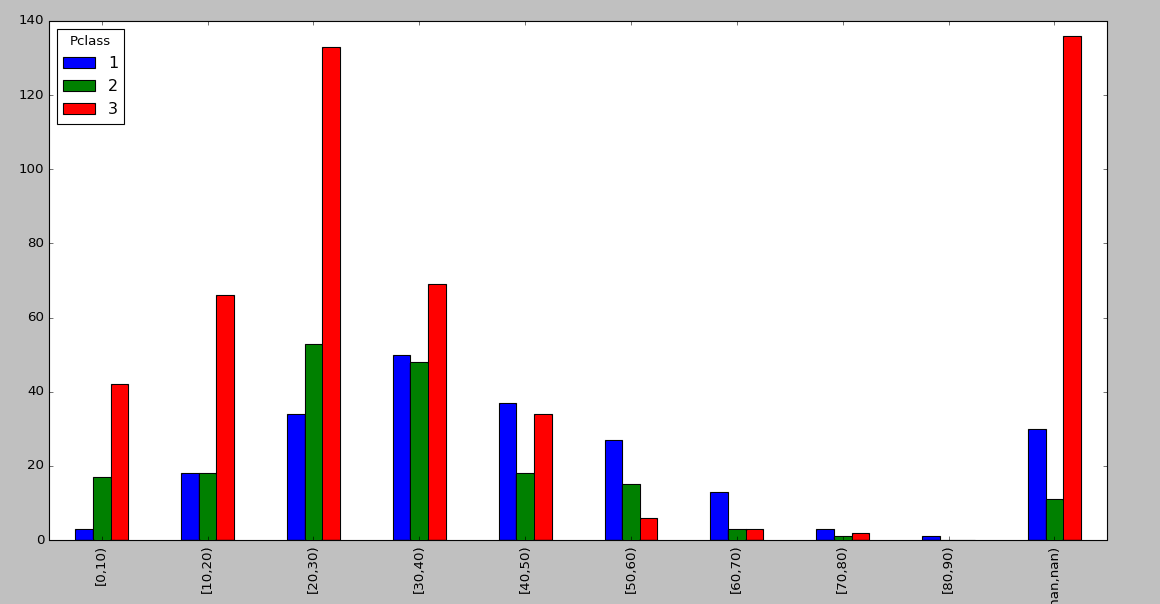lim = 10

def cla(n,lim):

return '[%.f ,%.f)'% (lim*(n//lim) , lim*(n//lim)+lim)

addone = Series([cla(s,lim) for s int.Age])

(groups['Name'].unstack()).plot(kind = ’bar’)

plt.show()09-028185
10-068558
04-09297
12-071018
08-04189
12-122840
02-252万+